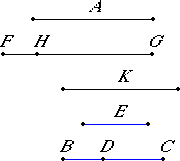# Proposition 90

To find the sixth apotome.

Set out a rational straight line A, and set out three numbers E, BC, and CD not having to one another the ratio which a square number has to a square number, and further let CB also not have to BD the ratio which a square number has to a square number.

X.6,Cor.

Let it be contrived that E is to BC as the square on A is to the square on FG, and BC is to CD as the square on FG is to the square on GH.X.6

Now since E is to BC as the square on A is to the square on FG, therefore the square on A is commensurable with the square on FG.

But the square on A is rational, therefore the square on FG is also rational. Therefore FG is also rational.

X.9

Since E does not have to BC the ratio which a square number has to a square number, therefore neither does the square on A have to the square on FG the ratio which a square number has to a square number, therefore A is incommensurable in length with FG.

X.6

Again, since BC is to CD as the square on FG is to the square on GH, therefore the square on FG is commensurable with the square on GH.

But the square on FG is rational, therefore the square on GH is also rational. Therefore GH is also rational.

X.9

Since BC does not have to CD the ratio which a square number has to a square number, therefore neither does the square on FG have to the square on GH the ratio which a square number has to a square number. Therefore FG is incommensurable in length with GH

X.73

And both are rational, therefore FG and GH are rational straight lines commensurable in square only. Therefore FH is an apotome.

I say next that it is also a sixth apotome.

V.22

Since E is to BC as the square on A is to the square on FG, and BC is to CD as the square on FG is to the square on GH, therefore, ex aequali, E is to CD as the square on A is to the square on GH.

X.9

But E does not have to CD the ratio which a square number has to a square number, therefore neither does the square on A have to the square on GH the ratio which a square number has to a square number. Therefore A is incommensurable in length with GH. Therefore neither of the straight lines FG nor GH is commensurable in length with the rational straight line A.

Now let the square on K be that by which the square on FG is greater than the square on GH.

V.19,Cor.

Since BC is to CD as the square on FG is to the square on GH, therefore, in conversion, CB is to BD as the square on FG is to the square on K.

X.9

But CB does not have to BD the ratio which a square number has to a square number, therefore neither does the square on FG have to the square on K the ratio which a square number has to a square number, therefore FG is incommensurable in length with K.

And the square on FG is greater than the square on GH by the square on K, therefore the square on FG is greater than the square on GH by the square on a straight line incommensurable in length with FG.

X.Def.III.6

And neither of the straight lines FG nor GH is commensurable with the rational straight line A set out. Therefore FH is a sixth apotome.

Therefore the sixth apotome FH has been found.

Q.E.F.

## Guide

This proposition is not used in the rest of the Elements.